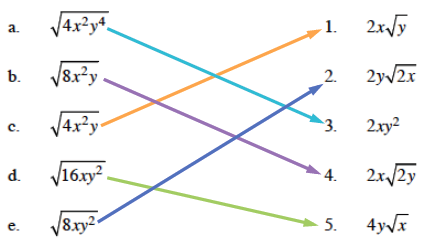### Home > CAAC > Chapter 13 > Lesson 13.RE1-S > Problem2-123

2-123.

Match the expressions on the left with their equivalent expressions on the right. Assume that all variables represent positive values. Be sure to justify how you know each pair is equivalent. Homework Help ✎

 a.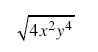1.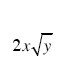b.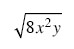2.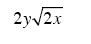c.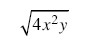3. 2xy2 d.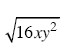4.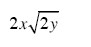e.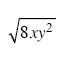5.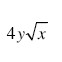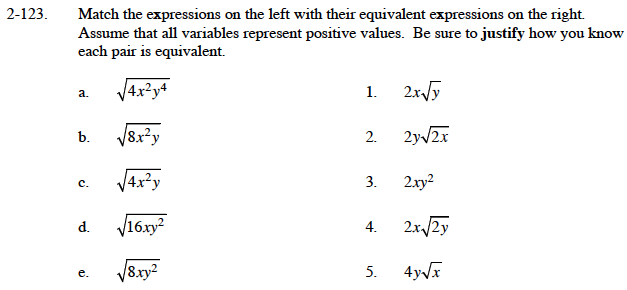Simplify the expressions in the right column. For example:

$\sqrt{8\textit{xy}^2} = \sqrt{4 \cdot 2 \cdot \textit{x} \cdot \textit{y}^2}=\sqrt{4} \cdot \sqrt{\textit{y}^2} \cdot \sqrt{2\textit{x}}$

Take the square roots of 4 and y2.